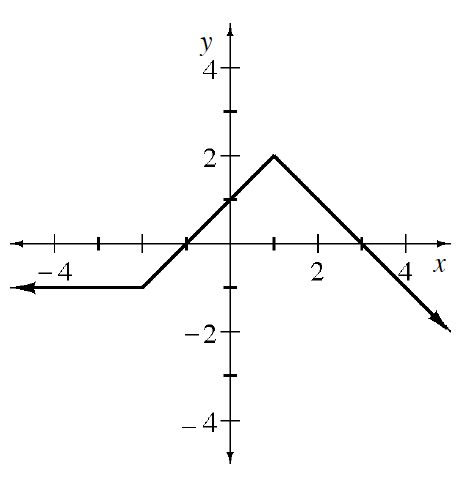### Home > APCALC > Chapter 1 > Lesson 1.3.1 > Problem1-106

1-106.

Using the graph of $y = f(x)$ at right, sketch the following transformations.

1. $y = −f(x)$

1. $y = f(x + 3)$

1. $y = f(x) − 2$

1. $y = | f ( x ) |$

Use the eTool below to view the graphs of the transformations.
Click the link at right for the full version of the eTool: Calc 1-106 HW eTool